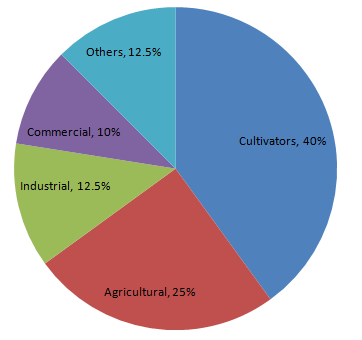# The percentages of various categories of workers in a state are given in the following table.

Question:

The percentages of various categories of workers in a state are given in the following table.

 Categoies Culti-vators Agricultural Labourers Industrial Workers Commercial Workers Others % of workers 40 25 12.5 10 12.5

Present the information in the form a pie-chart.

Solution:

We know:

Central angle of a component = (component value/sum of component values ×">× 360)

Here, total percentage of workers = 100

Thus, the central angle for each component can be calculated as follows:

 Category Percentage of workers Sector angle Cultivators 40 40/100 ×">× 360 = 144 Agricultural labourers 25 25/100 ×">× 360 = 90 Industrial workers 12.5 12.5/100 ×">× 360 = 45 Commercial workers 10 10/100 ×">× 360 = 36 Others 12.5 12.5/100 ×">× 360 = 45

Now, the pie chat representing the given data can be constructed by following the steps below:

Step 1 : Draw circle of an appropriate radius.

Step 2 : Draw a vertical radius of the circle drawn in step 1.

Step 3 : Choose the largest central angle. Here, the largest central angle is 144o. Draw a sector with the central angle 144o in such a way that one of its radii coincides with the radius drawn in step 2 and another radius is in its counter clockwise direction.

Step 4 : Construct other sectors representing other items in the clockwise direction in descending order of magnitudes of their central angles.

​Step 5 : Shade the sectors with different colours and label them, as shown as in figure below.​​